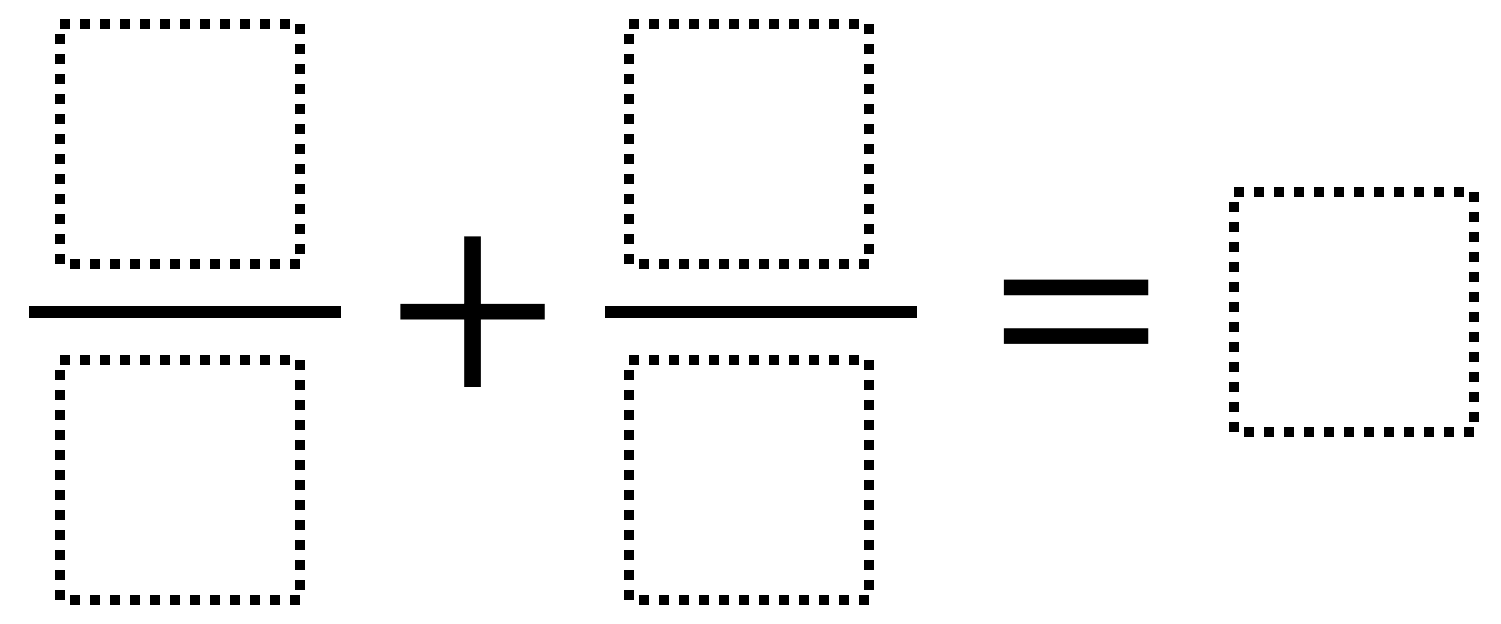Home > Grade 5 > Adding Fractions 6

# Adding Fractions 6

Directions: Use the digits 1 to 9, at most one time each, to make a true statement.### Hint

What fractions add together to make a whole number?

There are many answers. Here are three possibilities:
8/2 + 9/3 = 7
3/1 + 4/2 = 5
2/4 + 3/6 = 1

Source: Owen Kaplinsky

## Multiplying Decimals to Make a Whole Number Product

Directions: Using the digits 1 to 9, at most one time each, fill in the …

1.4/2 + 3/1 = 5

2.My students decided to do the inverse of their answers to create improper fractions that were equivalent to whole numbers and then add them up. We also had fun with figuring out other mixed numbers to equal wholes (see 9/6 below).

6/3 + 2/1 = 4
8/2 + 5/1 = 9
9/6 + 8/3 = 4 (this one we had to try several times to work out something with 9/6)
3/6 + 4/8 = 1

Working with my other classes later this week. If we come up with more I will add them.

•How fun! I want to try this one in my class. Mel Paradis, I’m not sure I understand what you mean about does 9/6 + 8/3 = 4. I found 9/6 + 8/3 = 4 not true if 8/3 = 16/6.
9/6 + 16/6 = 25/6 = 4 1/6. Did I miss something?